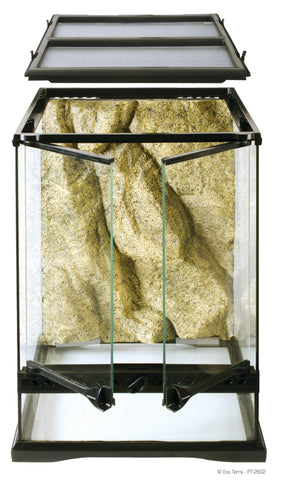# How many gallons is my enclosure? How do I figure out how much substrate that I need?

Common Enclosure Conversions
We here at The Bio Dude, pride ourselves on helping each kind of keeper,
from the extreme novice to the seasoned expert. Since we as reptile and pet owners never stop growing and evolving. Proper housing and figuring out which enclosure to purchase can be a little tricky- we are here to help.
You will find the most common enclosure conversions. You can find out how
much substrate is included in the kits by visiting The Dude’s website under
“Bioactive Kits”. From there choosing the kit that’s closets to your enclosure
size. Glass enclosures rounded up to the nearest whole number to give an accurate gallon conversion. L X W X H /231 is the formula used.
Dimensions are listed as LENGTH X WIDTH X HEIGHT8x8x8 = 3 gallons
8x8x12 = 4 gallons
12x12x12= 8 gallons
12x12x18= 12 gallons
18x18x12= 17 gallons
18x18x18= 26 gallons
18x18x24= 30 gallons
18x18x36= 50 gallons
24x18x12= 23 gallons
24x18x18= 33 gallons
24x18x24= 45 gallons
24x18x36= 67 gallons
36x18x12= 34 gallons
36x18x16= 45 gallons
36x18x18= 50 gallons
36x18x24= 67 gallons
36x18x36= 100 gallons
48x18x21= 75 gallons
• Josh Halter